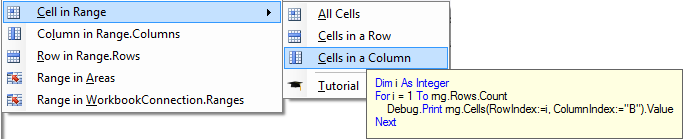﻿ Excel Range Cells VBA

# Using Excel Range Cells in VBA

 A B C 1 2 a b 3 c d 4 e f

The following sections explain how you can use worksheet or range object `.Cells` property. It begins with explaining two ways to select a cell. Next, three ways to loop over the cells collection are shown: all cells, in a certain row and a column. All examples refer to the worksheet image on the right with table a..f.

## Select a cell

The `.Cells` property returns a collection of cells. Which cells are to be included is determined by the RowIndex and ColumnIndex properties as explained below. There are three classes that support the Cells property:

• `Application.Cells`: cells on the active worksheet. This is what is assumed when Cells is called without specifying the range or worksheet.
• `Worksheet.Cells`: cells on the specified worksheet (not just the cells that are currently in use).
• `Range.Cells`: cells in the specified range.

There are two ways to identify a single cell in the Cells collection:

1. Select cell using row and column index
2. N-th item in the collection

### Select cell using row and column index

The Cells property has two arguments `RowIndex` and `ColumnIndex`

ExpressionValueComment
`Cells(2, 2)`aCell on active worksheet in row 2 and col 2
`Range("B2").Cells(2, 2)`dCell on row 2 and col 2 starting count from B2
`Range("B2:B3").Cells(2, 2)`dCount needs not be inside the specified range
`Range("B2:B3").Cells(2, "B")`dColumn character interpreted as number, e.g. "B" is always 2nd column
Note
Negative values in row or columnindex are not allowed.

### N-th item in the collection

When leaving out the second optional argument `ColumnIndex`, the Cells property returns the nth item. The order in which items get returned is breadth-first, so in the example table the cells with value a,b,c,...f.

ExpressionValueComment
`Range("B2:B3").Cells(2)`cThe second cell in the range
`Range("B2:B3").Cells(3)`eEven though the range only has two cells, ...
`Range("B2:C3").Cells(6)`f... it interprets as extra rows with the number of columns given in the range

## Loop over cells in Range

The `Cells` property is particularly useful because it makes it easy to iterate over a range. The image below shows the part of the Code VBA (download) menu that lets you insert the code fragment you require.### Loop over all cells in the range

The code below shows how you can loop over all cells in the range. Here it would print values a,b,c,d from the table at the top of the page.

``````Dim rng As Range: Set rng = Application.Range("B2:C3")
Dim cel As Range
For Each cel In rng.Cells
Debug.Print cel.Value
Next cel
``````

### Loop over cells in a row

The code below returns c and d. It knows it only has to obtain `rng.Columns.Count` values. It gets them from column 2 relative to the start of the range "B2", which is interpreted as row 3 on the worksheet.

``````Dim rng As Range: Set rng = Application.Range("B2:C3")
Dim i As Integer
For i = 1 To rng.Columns.Count
Debug.Print rng.Cells(RowIndex:=2, ColumnIndex:=i).Value
Next``````

### Loop over cells in a column

The code below returns b and d. It knows it only has to obtain `rng.Rows.Count` values. It gets them from column B relative to the start of the range "B2", which is interpreted as column C on the worksheet.

``````Dim rng As Range: Set rng = Application.Range("B2:C3")
Dim i As Integer
For i = 1 To rng.Cells.Rows.Count
Debug.Print rng.Cells(RowIndex:=i, ColumnIndex:="B").Value
Next``````